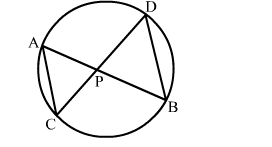# In a circle, two chords AB and CD intersect at a point inside the circle. Prove thatQuestion:

In a circle, two chords AB and CD intersect at a point inside the circle. Prove that

(a) $\triangle \mathrm{PAC} \sim \triangle \mathrm{PDB}$

(b) PA.PB = PC. PDSolution:

Given: AB and CD are two chords
To Prove:

(a) $\triangle \mathrm{PAC} \sim \triangle \mathrm{PDB}$

(b) $\mathrm{PA} . \mathrm{PB}=\mathrm{PC} . \mathrm{PD}$

Proof: In $\triangle \mathrm{PAC}$ and $\triangle \mathrm{PDB}$

$\angle \mathrm{APC}=\angle \mathrm{DPB}$ (Vertically Opposite angles)

$\angle \mathrm{CAP}=\angle \mathrm{BDP}$ (Angles in the same segment are equal)

By AA similarity-criterion $\triangle \mathrm{PAC} \sim \triangle \mathrm{PDB}$

When two triangles are similar, then the ratios of the lengths of their corresponding sides are porportional.

$\therefore \frac{\mathrm{PA}}{\mathrm{PD}}=\frac{\mathrm{PC}}{\mathrm{PB}}$

$\Rightarrow$ PA.PB $=$ PC.PD